# Oversimplified: Signals and Systems (5) – Time-Invariance, Linearity (Superposition) and ConvolutionL and TI are two distinct concepts. Convolution is a concept (straight from LTI), not a definition.

Convolution is one of the major topics in signal processing. It’s the immediate next step after complex numbers and linear algebra: you won’t get far without mastering convolution first.

Unfortunately, because the traditional approach of teaching signal processing assumed the audience doesn’t know linear algebra, they jumped to the definition of convolution instead of telling you what leads to it, then made you go through a bunch of exercises until you feel ‘comfortable’ with it.

Here are the common damages caused by teaching convolution as a definition rather than a concept:

• Many will overlook that any LTI system can be fully described as convolution with an impulse response. Very few can confidently tell you why without whipping out a mathematical proof.
• Many cannot tell linearity and time-invariance apart*.
• Supposedly ‘obvious’ connections between convolution to applications (e.g. reverberation and ghosting) won’t get noticed until explicitly taught.
• The course worked you through the painful and mindless ‘flip-and-drag’ method solely because the definition of convolution says so.

Understanding what ideas lead to the definition of convolution will help you spot intuitive applications and write it down correctly each time without hesitation. To prevent confusion caused by the common approach, I’ll go in the following order:

1. Time invariance
The ‘machinery’ (system) responds to the input sequence exactly the same way regardless of when they show up: the expected output is only delayed as much as the input is delayed, with no other alterations whatsoever.In laymen’s terms: if you shout at the cliff 5 minutes later than planned, you will hear the exact same echo exactly 5 minutes later than originally expected.Mathematically,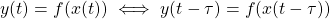. Looks trivial as you just replacedwith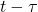, but if the system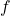changes with(aka time-variant), you have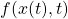. Responding to delayed input becomes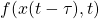, not that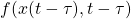you wished for. The relationship is not simple anymore!
2. Linearity (Superposition)
Superposition doesn’t care what kind of inputs you feed into it: It can be genuinely from multiple simultaneous sources, how you imagine the inputs could be broken down into, or even a data point coming from the future or past copy of itself.Superposition simply doesn’t have the concept of time. It provides the same treatment to each (additive) input components so that the pooled output will be the same as if the inputs were lumped (summed) together.Mathematically,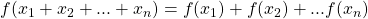. It doesn’t care what you have for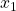,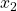, …, etc. You can have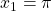,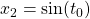,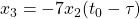, … and so on. I used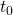to emphasize that it’s a snapshot (data point) which doesn’t generalize across time likedoes.
3. LTI: Superposition over different delays made possible by time-invariance
Because linearity by itself doesn’t have any memory (or any concept of time), superposition only applies snapshot-wise (reacting to the set of input components it sees at the same time).This mean superposition alone does not apply across different times. In other words, the input components are instantaneous points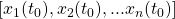, not functions of time!To generalize superposition to component input functions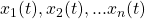working across different times, i.e. taking a linear combination of functions instead of just points, you’ll need the system to stay fixed (time invariant) so the later inputs are treated by the same system as the earlier ones.

This is why LTI systems are often desirable: the time-invarance property (TI) allows a linear system (L) to accept a delayed copy of a function (e.g.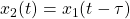) as one of the legitimate inputs for superposition.

4. Express LTI mathematically: convolution
Let’s call a delayed copy of a function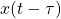echo. For each echo (at delay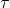), an LTI system assigns a gain/attenuation factor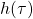. The output is all the gained/attenuated echos combined (pooled) together by a sum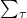or an integral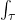. Write it out mathematically: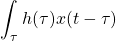In linear algebra speak, the output of the LTI is a linear combination of different echos (functions at different shifts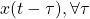), weighted by the impulse response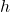.

There is no way you can remember the definition of convolution wrong if you think of it as weighting each echo before lumping them together! It’s immediately obvious thatis the running variable (to sum over), samples the impulse response, and is the delay offset because it represents (indexes) each echo!

At this point, you have already noticed convolution directly represents reverberation (multiple echos in a room) and ghosting (multi-path reflection has multiple copies of the same signal attenuated and arrive at the receiver with different delays).

* Bad enough that the pioneers of adaptive filters had to debate in their textbooks whether an adaptive (linear combiner) filter (an advanced topic) is linear or not. Adaptive filters are instantaneously linear, but intentionally very time-variant!

If the filter coefficients never change with time (time-invariant), superposition still applies! The only catch is that with adaptive filters, you cannot consider delayed copies as input components for the purpose of superposition because a time-varying system will treat each delayed copy differently.

Think of it as 4 different linear systems (one for each time instance): only if all 4 systems are identical, you can pool the results together and pretend it’s one system. Individually they are linear systems, but without the time-invariant property, you cannot bunch them together and pretend it’s one system at all times.